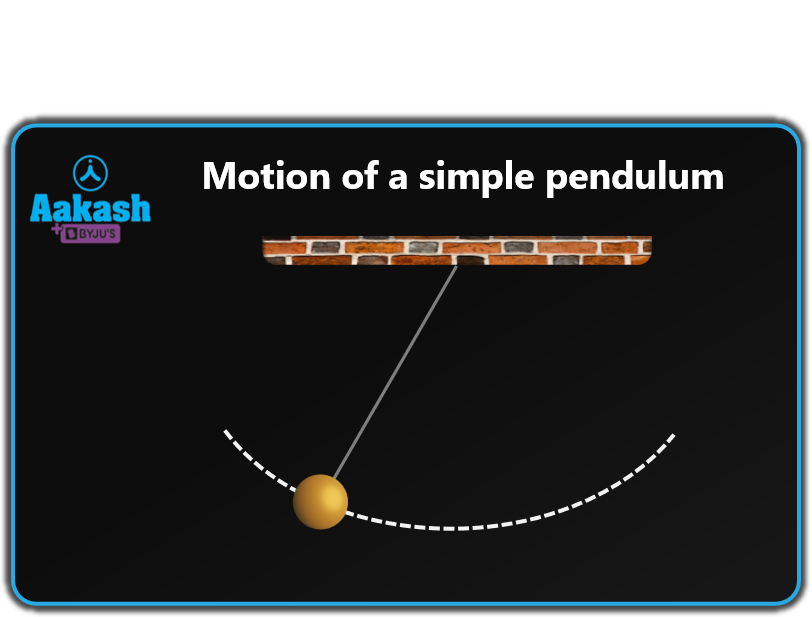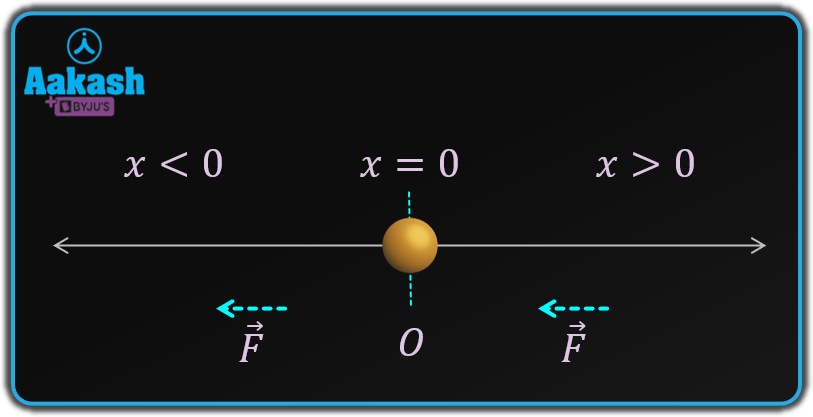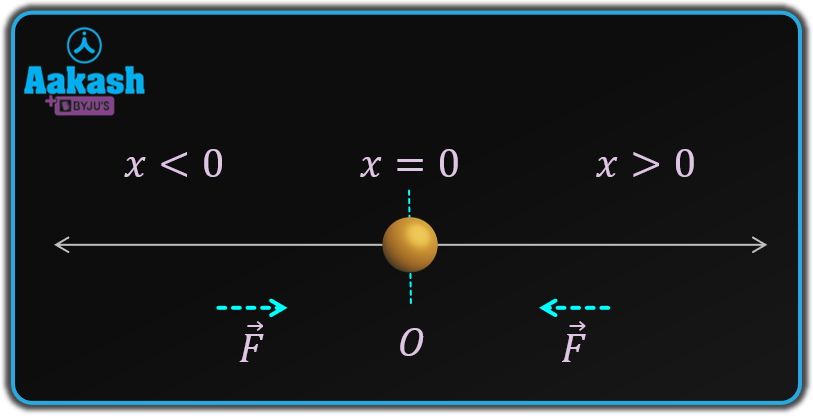• Call Now

1800-102-2727•

## Oscillatory motion, Simple harmonic motion as Oscillatory motion, Practice problems, FAQs

Have you seen the bobs in the science laboratory? When a freely hanging bob is pushed, it tends to move a particular distance on one side, return to equilibrium, then move the same distance on the other side to shift its position from equilibrium. This cycle continues until all the energy is used up. As a result, motion of a bob that is suspended freely by a string is called oscillatory motion. Let's understand the oscillatory motion in detail!

Table of content

• Oscillatory motion
• Types of oscillatory motion
• Expression for oscillatory motion
• Simple harmonic motion as Oscillatory motion
• Practice problems
• FAQs

## Oscillatory motion

The to-and-fro motion of an object relative to its mean position is referred to as oscillatory motion. The oscillating pendulum is an example of oscillatory motion.In a frictionless environment, the object may theoretically oscillate continuously, but in the real world, this is not possible, thus the body must come to equilibrium. In the mechanical world, the movement of springs and the vibration of strings also refers to oscillatory motion.

It is important to distinguish oscillatory motion from periodic motion. In oscillatory motion, objects repeat their movement over a set position, whereas in periodic motion, objects repeat their motion after a fixed duration or period of time.

## Types of oscillatory motion

Oscillatory motions come in two types: linear oscillatory motion and circular oscillatory motion.

In linear oscillatory motion, an object goes up and down or left and right. Few example of the oscillatory are -

• String vibrations on musical instruments
• Fluid movement in a U-tube column
• Ships and other large boats floating in the sea

In the circular oscillatory motion the object moves from left to right, but it does so in a circular motion. The example of circular oscillatory motion are -

• The pendulum's motion in a watch
• The swing's motion
• a object on a rope hanging from a nail

## Expression for oscillatory motion

Let the situation when a particle is being acted upon by a force , where n is an integer, Now there are two cases possible,

If n is an even integer i.e. n=0, 2, 4...... : In this case the force will always be applied along the -ve x-axis for both positive and negative body displacements as shown in figure below. This demonstrates that the body cannot oscillate about its mean position in a to-and-fro motion. Hence for even integral values of n, the particle's motion is not oscillatory.If n is an odd integer i.e. n=1, 3, 5...... : In this case, the force will be applied to the positive x-axis for the negative displacement (-x).

$⇒F\propto -\left(-x{\right)}^{3}$ Or $F\propto +\left(x{\right)}^{3}$

Similar, the force will be applied to the negative x-axis for positive displacement (+x).

$⇒F\propto -\left(+x{\right)}^{3}$ Or $F\propto -\left(x{\right)}^{3}$

As a result, the force will always work in the opposite direction of the displacement and tend to return the body to its mean position (x = 0). The body will move back and forth about x = 0. Hence for the odd integral values of n, the particle will move in an oscillatory fashion.## Simple harmonic motion as Oscillatory motion

Simple harmonic motion is the simplest form of oscillatory motion. A particle is said to be in simple harmonic motion when it oscillates around a fixed point such that its net force is proportional to its displacement from the fixed point and its direction is toward the mean position. I.e.

$F\propto -x$
Or
$F=-kx$

Where k= force constant and x is the displacement from the mean position and negative sign shows that the force is acting against the displacement.The term "mean position" refers to the fixed point around which a particle oscillates. Extreme position is defined as the greatest distance the particle can travel from mean position. A motion's amplitude is defined as the difference between its mean and extreme positions. The time period of simple harmonic motion is the period of time needed to complete one oscillation.

The general equation of the simple harmonic motion is given as,

$\mathbit{x}=\mathbit{A}\mathbf{sin}\left(\mathbit{\omega }\mathbit{t}+\mathbit{\varphi }\right)$

Where, A= amplitude of SHM

$\omega =$ angular frequency of SHM

$\varphi =$initial phase

## Practice problems

Q1. The equation $F=-k{x}^{n}$ describes a force acting on a moving object. The motion will be oscillatory at what value of n?

a) odd
b) even
c) either even or odd
d) Any real value

Answer. If motion is oscillatory, the force on the body should be directed toward the mean position. Now if n is integer the force will only operate in one direction and the motion will be rectilinear.

And the motion will be in the opposite direction from the direction of x if n is odd. Hence option (a) is correct.

Q2. A particle's displacement is given by the equation , where x is in meters and t is in seconds.What is the amplitude of the motion?

Answer. On comparing with the general equation of SHM

$\mathbit{x}=\mathbit{A}\mathbf{sin}\left(\mathbit{\omega }\mathbit{t}+\mathbit{\varphi }\right)$

We have Amplitude A=2.0 m

Q3. The oscillatory motion of a particle is described by the equation what is the displacement of particle at t=3 s from its mean position?

Put t=3 in the equation

x=-2.1213 cm

Hence particle is 2.1213 cm away from the mean position in negative x- direction.

Q4. Equation represents an oscillatory motion of a particle. Find the frequency and time period.

Answer. On comparing with the general equation of SHM

$\mathbit{x}=\mathbit{A}\mathbf{sin}\left(\mathbit{\omega }\mathbit{t}+\mathbit{\varphi }\right)$

We get

$\omega =6\pi$

The frequency of Oscillatory motion is

The time period

Hence frequency is 3 Hz and time period is 0.33 s.

## FAQs

Q1. Every oscillatory motion is periodic motion but is the inverse not true?
Periodic motion is motion in which a particle repeats its motion after a fixed interval for example rotation of earth. And for motion to be oscillatory it should move to and fro about a fixed point so it will be periodic by default. Hence we can conclude every oscillatory motion is periodic but the inverse is not true.

Q2. Every simple harmonic motion is an oscillatory motion but the inverse is not true. Why?
In simple harmonic motion the force is directly proportional to the displacement from the mean position i.e. $F\propto -x$ which is a particular case of the oscillatory motion with value of n=1. So every SHM is an oscillatory motion. If n=2 then motion is not SHM but it is oscillatory so inverse is not true.

Q3. What is the relation between frequency and time period?
Time period is inversely proportional to the frequency. If the time period is more, frequency will be higher and vice versa.

Q4. In which position the force on the particle is maximum in an oscillatory motion?
Force is maximum at the extreme position in an oscillatory motion and zero at the mean position.Talk to our expert
Resend OTP Timer =
By submitting up, I agree to receive all the Whatsapp communication on my registered number and Aakash terms and conditions and privacy policy